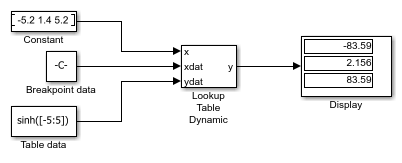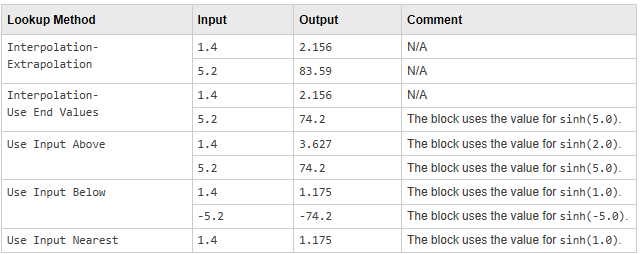This example shows how to use the Lookup Table Dynamic block to approximate the `sinh` function. The breakpoint data is given by the vector `[-5:5]` and the table data is given by the vector `sinh([-5:5])`. The input `x` is provided by the Constant block as a 1-by-3 vector containing values that are below, within, and above the breakpoint data values.